• 矩阵乘法并行化与实现
说到矩阵乘法，最先想到的就是用两个for循环，循环矩阵A的行再循环矩阵B的列，从而实现矩阵A与B的相乘。
（1）下面是串行算法的实现代码：
#include<stdlib.h>
#include<stdio.h>
typedef struct
{
int** mat;		//指向指针的指针
int row,col;
}matrix;
void initial(matrix &M,int row,int col)
{
int i,j = 0;
M.mat = (int **)malloc(row*sizeof(int *));		//分配列空间
for (i = 0; i < row; i++)
M.mat[i] = (int *)malloc(col*sizeof(int));	//分配行空间
M.row = row;
M.col = col;
}
void initValue(matrix &M, int row, int col)
{
int i, j;
initial(M,row,col);
for (i = 0; i < row; i++)
{
printf("请输入第%d行的数据:",i);
for (j = 0; j < col; j++)
scanf("%d",&M.mat[i][j]);
}
}
void Matrix_Multiply(matrix &A,matrix &B,matrix &C)
{
int i,j,k;
for(i=0;i<A.row;i++)
{
for(j=0;j<B.col;j++)
{
C.mat[i][j]=0;		//矩阵C需要初始化为0,否则会输出负值
for(k=0;k<B.row;k++)
C.mat[i][j]+=A.mat[i][k]*B.mat[k][j];
}
}
}
void main()
{
matrix A,B,C;
int row_a,col_a,row_b,col_b;
printf("请输入矩阵A的行数,列数:");
scanf("%d,%d",&row_a,&col_a);
printf("请输入矩阵B的行数,列数:");
scanf("%d,%d",&row_b,&col_b);
if(row_a<0||col_a<0||row_b<0||col_b<0||col_a!=row_b)
printf("输入数据有误!");
else
{
printf("矩阵A初始化\n");
initValue(A,row_a,col_a);
printf("矩阵B初始化\n");
initValue(B,row_b,col_b);
initial(C,row_a,col_b);
Matrix_Multiply(A,B,C);
printf("矩阵A*B的结果:\n");
for(int i=0;i<C.row;i++)
{
for(int j=0;j<C.col;j++)
printf("%d ",C.mat[i][j]);
printf("\n");
}
}
}
（2）并行算法采用的是我们在计算时经常使用的行列相乘法，即把将A按行（A1 A2......）分配到A.row个处理器上，后是通过B（B1 B2......）的移位从而实现A的每一行与B的每一列相乘，从而实现并行化并行算法的实现代码：
//#include"stdafx.h"
#include<stdlib.h>
#include<stdio.h>
#include<windows.h>
typedef struct
{
int** mat;		//指向指针的指针
int row,col;
}matrix;
typedef struct
{
int	a;		//A的行数
int b;		//B的列数
}parameter;
matrix A,B,C;
HANDLE* h=(HANDLE* )malloc(sizeof(HANDLE)*A.row);
void initial(matrix &M,int row,int col)
{
int i,j = 0;
M.mat = (int **)malloc(row*sizeof(int *));		//分配列空间
for (i = 0; i < row; i++)
M.mat[i] = (int *)malloc(col*sizeof(int));	//分配行空间
M.row = row;
M.col = col;
}
void initValue(matrix &M, int row, int col)
{
int i, j;
initial(M,row,col);
for (i = 0; i < row; i++)
{
printf("请输入第%d行的数据:",i);
for (j = 0; j < col; j++)
scanf("%d",&M.mat[i][j]);
}
}
void Multiply(void *n)		//i是A的行数
{
int i;
parameter p=*((parameter *)n);
C.mat[p.a][p.b]=0;
for(i=0;i<A.col;i++)
{
C.mat[p.a][p.b]+=A.mat[p.a][i]*B.mat[i][p.b];
}
}
void Parallel_MatrixMultiply(matrix &A,matrix &B,matrix &C)
{
int i,j;
parameter* p=(parameter* )malloc(sizeof(parameter)*A.row);
int *tag=(int* )malloc(sizeof(int)*A.row);
for(i=0;i<B.col;i++)
tag[i]=i;
for(i=0;i<B.col;i++)
{
for(j=0;j<A.row;j++)
{
p[j].a=j;
p[j].b=tag[(i+j)%A.row];		//B移位的过程
0,
&p[j],		//不能直接传i
0,
NULL);
printf("创建线程\n");
}
}
}
void main()
{
int row_a,col_a,row_b,col_b;
printf("请输入矩阵A的行数,列数:");
scanf("%d,%d",&row_a,&col_a);
printf("请输入矩阵B的行数,列数:");
scanf("%d,%d",&row_b,&col_b);
if(row_a<0||col_a<0||row_b<0||col_b<0||col_a!=row_b)
printf("输入数据有误!");
else
{
printf("矩阵A初始化\n");
initValue(A,row_a,col_a);
printf("矩阵B初始化\n");
initValue(B,row_b,col_b);
initial(C,row_a,col_b);
Parallel_MatrixMultiply(A,B,C);
WaitForMultipleObjects(A.row,h,TRUE,INFINITE);
printf("矩阵A*B的结果:\n");
for(int i=0;i<C.row;i++)
{
for(int j=0;j<C.col;j++)
printf("%d ",C.mat[i][j]);
printf("\n");
}
}}
展开全文• ## 矩阵乘法的并行化算法讨论

万次阅读 多人点赞 2015-09-26 13:28:14
另一方面，矩阵乘法同时也是并行计算领域常常被用来作为范例的一个话题。它的特点是首先计算量可能相当大，适合利用并行实现来提高效率。其次，它所使用的各种数据之间（矩阵中的元素）没有相互依赖性，可以充分使用...
矩阵乘法是线性代数里面会讲到的一种非常基础、也十分普遍的计算规则。另一方面，矩阵乘法同时也是并行计算领域常常被用来作为范例的一个话题。它的特点是首先计算量可能相当大，适合利用并行实现来提高效率。其次，它所使用的各种数据之间（矩阵中的元素）没有相互依赖性，可以充分使用并行处理的计算资源。串行实现根据线性代数的基本知识，m × l 的矩阵A，乘以一个大小为 l × n 的矩阵B，将得到一个 m × n 的矩阵C=A×B，其中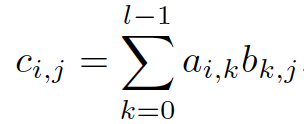下图是用图示来表示的这种计算规则：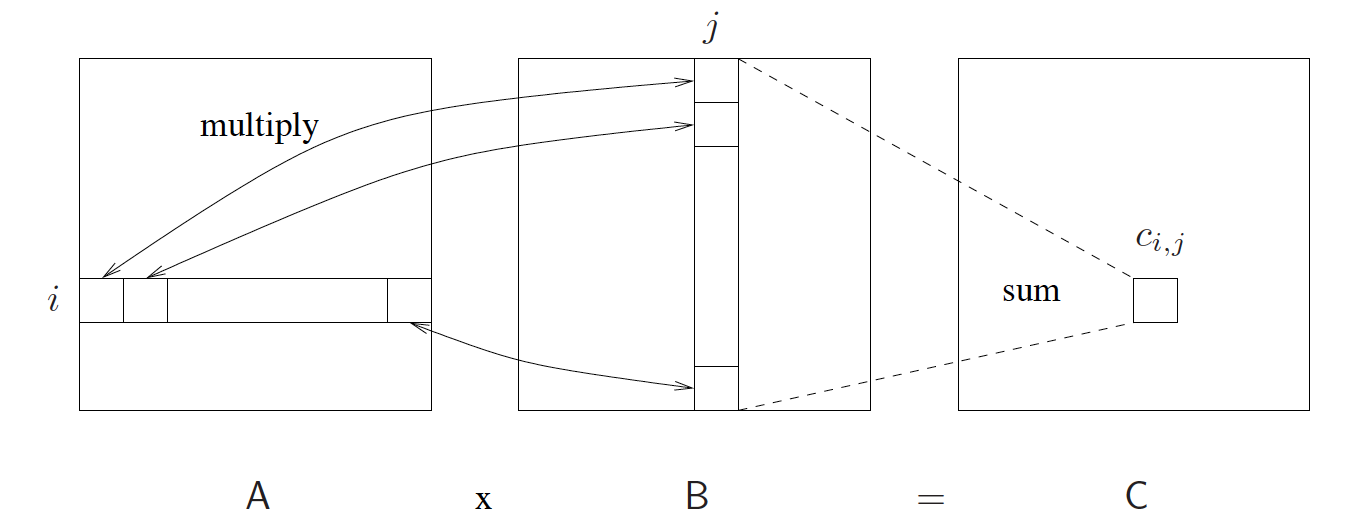为了方便讨论，我们可以不是普遍性地假设有所矩阵的大小都是 n × n 的，下面就是串行实现的矩阵乘法的代码：int A[n][n], B[n][n], C[n][n];
...
for(i=0;i<n;i++){
for(j=0;j<n;j++){
C[i][j]=0;
for(k=0;k<n;k++)
C[i][j]+=A[i][k]*B[k][j];
}
}
易见，这个算法的计算复杂度为O(n^3)。基本并行实现的讨论正如前面所讲的，矩阵相乘过程中，结果矩阵C中的每个元素都是可以独立计算的，即彼此之间并无依赖性。所以如果采用更多的处理器，将会显著地提高矩阵相乘的计算效率。对于大小为n × n 的矩阵，加入我们有n个处理器，那么结果矩阵中的每一行，都可以用一个处理器来负责计算。此时，总共的并行计算步数为 O(n^2)。你可以理解为在串行实现的代码中，最外层的循环 for(i=0;i<n;i++) 被分别由n个处理器来并行的执行，而每个处理需要完成的任务仅仅是内部的两层循环。如果采用n^2个处理器，那么就相当于结果矩阵中的每个元素都由一个处理器来负责计算。此时，总共的并行计算步数为 O(n)。你可以理解为在串行实现的代码中，最外面的两层循环 被分解到n^2个处理器来并行的执行，而每个处理需要完成的任务仅仅是内部的一层循环，即for(k=0;k<n;k++)。更进一步，如果有n^3个处理器，那么即使最内层的循环for(k=0;k<n;k++)也有n个处理器在并行的负责。但是最终的求和运算，我们需要一个类似reduction的操作，因此最终的计算复杂度就是O(log n)。当然，你一定会想到的是，实际中，通常并不可能有像矩阵元素那么多的处理器资源。这时我们该怎么做。对于一个大小为n × n 的大矩阵A，我们其实可以把它切分成s^2个子矩阵Ap,q，每个子矩阵的大小为 m × m，其中 m = n / s，即0 <= p, q < s。对于两个大矩阵A和B，现在我们有：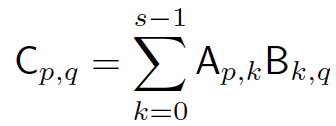用图示表示则有：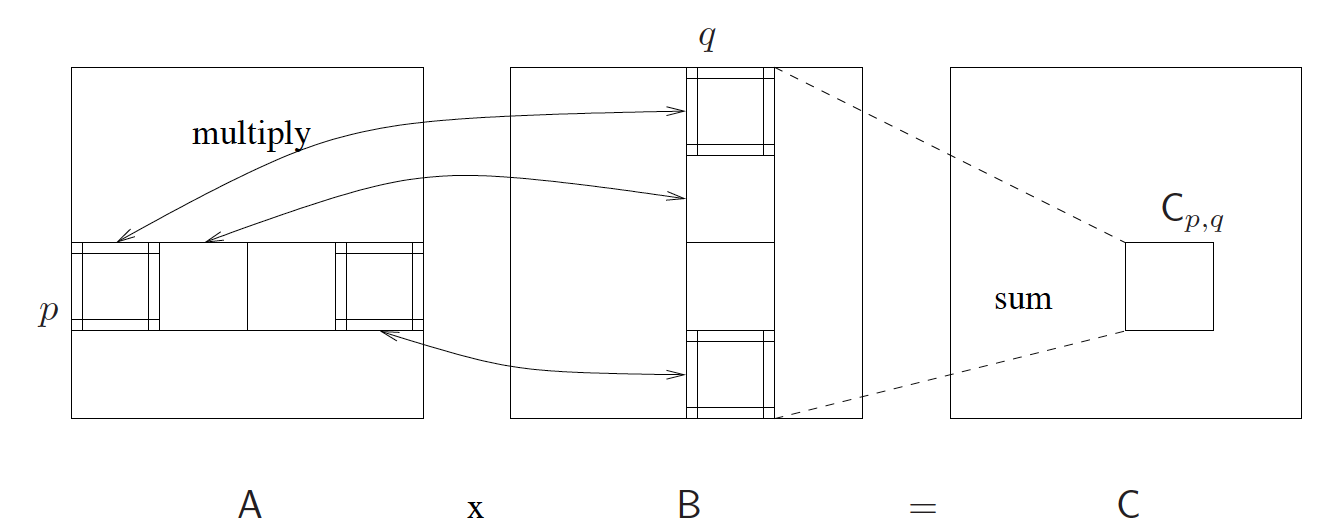Cannon算法著名的Cannnon算法使用一个由s^2个处理器组成的二维网孔结构（mesh），而且这个mesh还是周边带环绕的（The processors are connected as a torus）。处理器Processor (i,j) （我们用它来表示位于位置(i,j)处的处理器）最开始时存有子矩阵Ai,j和Bi,j。随着算法的进行，这些子矩阵会向左或向上位移。如下图所示：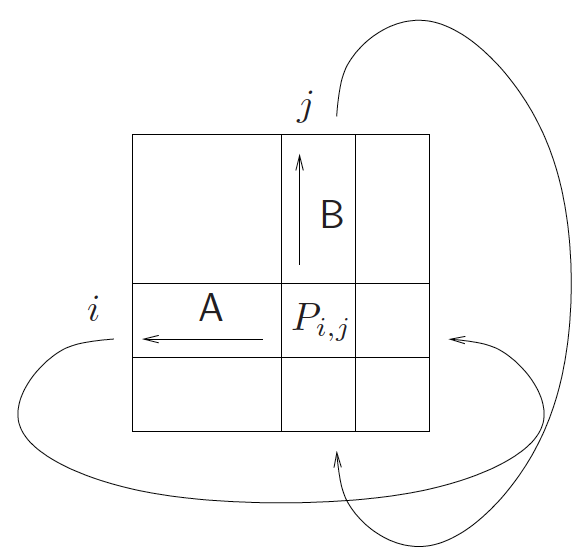这个算法的根本出发点是在处理器阵列中，要合理分布两个待乘的矩阵元素。由乘积公式可知，要在处理单元 P(i,j)中计算乘积元素C(i,j)，必须在该单元中准备好矩阵元素A(i,s)和B(s,j)。但是如果我们像下图那样分布矩阵元素，我们在计算C(i,j)时所需的元素显然是不足够的，但是可以通过向上循环位移B的元素，并向左循环位移A的元素，来获取合适的成对的矩阵元素。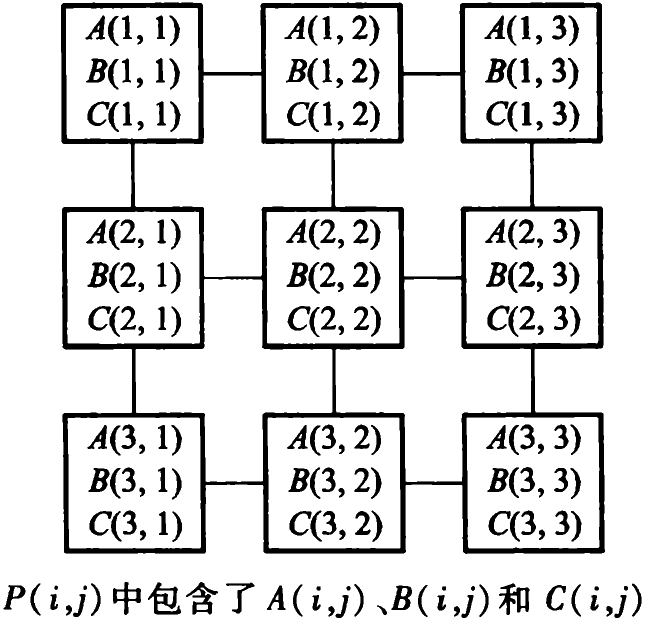Cannnon算法的具体流程：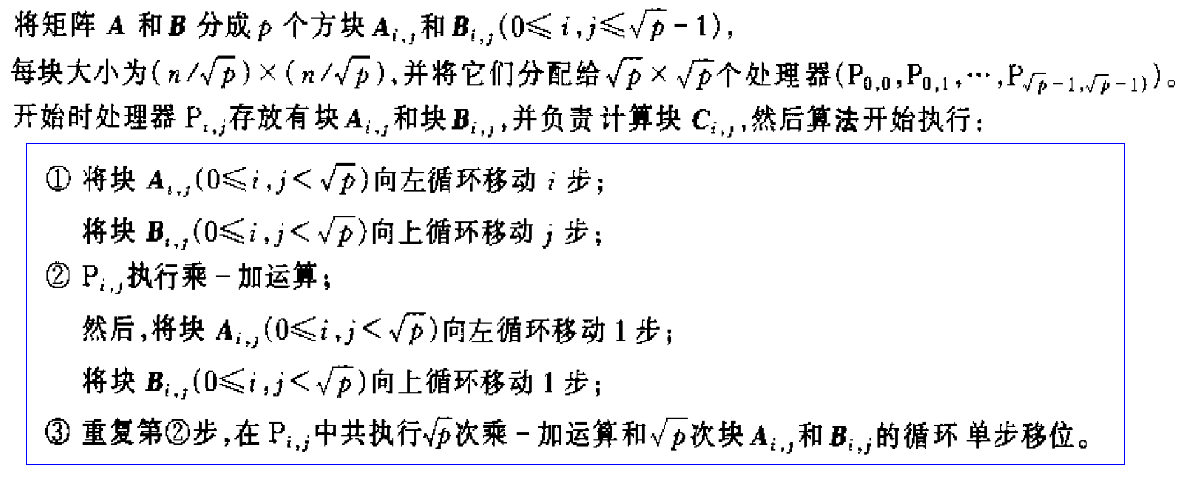下面是矩阵位移的一个示例，其中s=3；显然，算法的复杂度 t(n)=O(n)， p(n) = n^2，w(n) = O(n^3)，所以是成本最佳的。---------------------------------------------参考文献与推荐阅读材料【1】陈国良，并行算法的设计与分析（第3版），高等教育出版社，2009【2】矩阵计算并行算法（百度文库地址：http://wenku.baidu.com/view/d64ba9b4b14e852458fb57fc.html）（本文完）
展开全文Cannon算法
• ## 矩阵乘法并行优化

千次阅读 2016-01-13 15:26:16
• 在嵌套循环中，如果外层循环迭代次数较少时... 下面以矩阵乘法作为例子来讲述如何将嵌套循环并行化，以满足上述扩展性和负载平衡需求。  一个串行的矩阵乘法的函数代码如下： /** 矩阵串行乘法函数  @param in

在嵌套循环中，如果外层循环迭代次数较少时，如果将来CPU核数增加到一定程度时，创建的线程数将可能小于CPU核数。另外如果内层循环存在负载平衡的情况下，很难调度外层循环使之达到负载平衡。
下面以矩阵乘法作为例子来讲述如何将嵌套循环并行化，以满足上述扩展性和负载平衡需求。
一个串行的矩阵乘法的函数代码如下：
/** 矩阵串行乘法函数
@param    int *a - 指向要相乘的第个矩阵的指针
@param    int row_a - 矩阵a的行数
@param    int col_a - 矩阵a的列数
@param    int *b - 指向要相乘的第个矩阵的指针
@param    int row_b - 矩阵b的行数
@param    int col_b - 矩阵b的列数
@param    int *c - 计算结果的矩阵的指针
@param    int c_size - 矩阵c的空间大小（总元素个数）
@return   void - 无
*/
void Matrix_Multiply(int *a, int row_a, int col_a,
int *b, int row_b,int col_b,
int *c, int c_size)
{
if ( col_a != row_b || c_size < row_a * col_b )
{
return;
}

int i, j, k;
//#pragma omp for private(i, j, k)
for ( i = 0; i < row_a; i++ )
{
int row_i = i * col_a;
int row_c = i * col_b;
for ( j = 0; j < col_b; j++ )
{
c[row_c + j] = 0;
for ( k = 0; k < row_b; k++ )
{
c[row_c + j] += a[row_i + k] * b[k * col_b + j];
}
}
}
}
如果在外层循环前加上OpenMP的for语句时，它就变成了一个并行的矩阵乘法函数，但是这样简单地将其并行化显然无法满足前面所述的扩展性需求。
其实可以采用一个简单的方法将最外层循环和第2层循环合并成一个循环，下面便是采用合并循环后的并行实现。

void Parallel_Matrix_Multiply(int *a, int row_a, int col_a,
int *b, int row_b,int col_b,
int *c, int c_size )
{
if ( col_a != row_b )
{
return;
}

int i, j, k;
int index;
int border = row_a * col_b;

i = 0;
j = 0;
#pragma omp parallel private(i,j,k) num_threads(dtn(border, 1))
for ( index = 0; index < border; index++ )
{
i = index / col_b;
j = index % col_b;

int row_i = i * col_a;
int row_c = i * col_b;

c[row_c+j] = 0;
for ( k = 0; k < row_b; k++ )
{
c[row_c + j] += a[row_i+k] * b[k*col_b+j];
}
}
}
从上面代码可以看出，合并后的循环边界border = row_a * col_b;即等于原来两个循环边界之积，然后在循环中计算出原来的外层循环和第2层循环的迭代变量i和j，采用除法和取余来求出i和j的值。
需要注意的是，上面求i和j的值必须要保证循环迭代的独立性，即不能有循环迭代间的依赖关系。不能将求i和j值的过程优化成如下的形式：
if ( j == col_b )
{
j = 0;
i++;
}
// …… 此处代表实际的矩阵乘法代码
j++;
上面这种优化，省去了除法，效率高，但是只能在串行代码中使用，因为它存在循环迭代间的依赖关系，无法将其正确地并行化。

展开全文border parallel matrix c 优化MapReduce PageRank
•c语言 算法优化
• GPU并行加速矩阵乘法，有详细的程序、结果及分析
• 参考了网上搜索的Delphi并行代码，修改后实现矩阵乘法并行计算。 源码 unit UnitTest; interface uses Windows, Messages, SysUtils, Variants, Classes, Graphics, Controls, Forms, Dialogs, Grids, ...
• 基于MPI并行方法实现矩阵乘法 目录 1. 实验目的 3 2. 实验环境 4 3. 实验内容 4 3.1. 实验题目 4 3.2. 实验过程 5 3.2.1. 集群使用 5 3.2.2. 源码及解析 5 3.3. 执行时间截图 12 3.3.1. 基准程序...
• 设两个矩阵A和B，大小分别为M * N 和 N * P， 如果C = A * B， 则C的大小为M * P。 矩阵算法的算法表示，伪代码如下： for (i = 0; i ; ++i){ for (j = 0; j ; ++j){ C[i][j] = 0; for (k = 0; k ; ++k){ ...算法 parallel 性能优化 c cache
• 并行算法设计与分析课程总结 第三讲：稠密矩阵乘法 Cannon 乘法 Fox 乘法 Systolic乘法 脉动阵列 dns（CC）乘法
• 并行矩阵乘法器 它使用英特尔 SIMD 内在函数和 OpenMP 执行高度并行化矩阵乘法。 它比 naïve 版本快 45 倍（1.2 gigaFLOPS 增加到 55 gigaFLOPS）。 我在没有骨架的情况下用 C 写了这个。C
• C矩阵的大小为M * P，我们可以将C的计算下平均分配到每个核心上，即每个核分配ceil(M*P/CORE_NUM)个计算任何，即将上面的第一和第二层并行化。 首先将C转换成一维的数组T[M*P] , 则C[i][j] = T[i * M + j], 反...
•mpi
• ## CUDA编程（九）并行矩阵乘法

万次阅读 多人点赞 2016-04-09 17:44:16
在之前我们一直围绕着一个非常简单的求立方和的小程序学习CUDA，不过这个立方和的小程序没有什么实际意义，这篇博客我们用CUDA并行矩阵乘法，问题也比较简单，基于上一个立方和程序的经验，完成这个程序也不算太难...cuda GPU加速 计算机视觉 优化
• /*Function:C++实现并行矩阵乘法;Time: 19/03/25;Writer:ZhiHong Cc;*/运行方法：切到工程文件x64\Debug文件下，打开命令行，输入以下指令：mpiexec -n N Project.exe NUM// N代表开启进程数量，NUM代表矩阵规模大小...
• ## OpenMP矩阵乘法实现

千次阅读 2015-05-21 16:10:43openmpPthreadsc++ eclipse parallel matrix
• 算法简介 算法流程图 算法设计方法和模式任务划分：根据矩阵乘法公式中的累加计算的可分离性，将参与计算的两个矩阵分解成p个小矩阵块(共有p个计算节点)，每个节点只进行局部的小矩阵乘法，最终计算结束后将局部的...i
• 读的一篇论文的总结（4）矩阵分解的主要工作是利用分布式编程技术将矩阵分解算法并行化矩阵分解算法矩阵分解技术的目标是，对每个用户和项目构建一个向量因子模型，由k维向量表示，其中每个分量代表用户在一个因子...大数据 算法 分布式 hadoop
• 矩阵乘法并行化基本都是用加农算法，但是在共享内存的情况下，我觉得加农并没有优势。 加农保证了在每个变量全局单副本的情况下，并行度的提升。在共享内存时，没有变量复制的成本，所以直接使用带状划分可以避免...
• 《OPENCL异构并行计算》中讲了如何利用OPENCL进行矩阵乘法运算，并给出了使用局部存储器优化、使用向量加载指令以及一个工作项同时计算多个输出的例子，这里对其进行简单分析opencl
• 使用共享内存的并行稀疏矩阵乘法的Julia库。 该库实现了SharedSparseMatrixCSC和SharedBilinearOperator类型，以使其易于在共享内存系统上并行与稀疏矩阵相乘。 安装 要安装，只需打开Julia提示并致电 Pkg.clone(...Julia
• ## openmp矩阵乘法

千次阅读 2019-06-27 15:41:04
目录 ...初始三个double矩阵matrix_a,matrix_b和result，矩阵的行和列可以根据数据量大小自行调整。数组中的值使用c++11中的random类随机生成0到1之间的double值。 程序计时。使用c++11中的...openmp
• 用java编写两个n阶的方阵A和B的相乘程序，结果存放在方阵C中，其中乘法用for编译制导语句实现并行化操作，并调节for编译制导中schedule的参数，使得执行时间最短。 方阵A和B的初始值如下：java runnable 线程
•   本次实验分别使用串行算法、Cache优化算法、SSE编程和分片策略算法实现了矩阵乘法运算，实验采用同一个样本，即矩阵大小为512个元素，元素值为由时间生成的随机数，每个算法对此样本运行十次，并记录每次运行...SIMD编程...